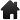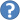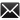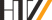≡ START First steps Deutsch Plane and sphere : Spherical triangles

From four input quantities of a spherical triangle all other quantities are computed, optionally including an . If two solutions exist, both are computed.

Angle unit    Note

QuantityValue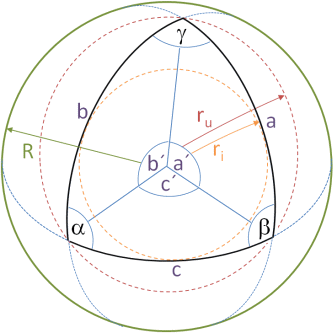A spherical triangle is determined by four quantities, usually by the radius of the sphere R and three further quantities. If the radius of the sphere is not specified, the computation will be successful only in rare cases. All angles α,β,γ of the triangle must be less than π = 180° = 200 gon . The triangle is not always uniquely determined. In non-unique cases both solutions will be displayed.

If the quantities a,b,c,s,ru,ri are terrestrial arc lengths in m or km, we recommend the radius R=6371000 or 6371.
If these quantities are specified or desired as angles in degrees, the radius should be R=57.2957795 etc.

Did you know? Blue-grounded input areas may be left blank.

≡ START First steps Deutsch In the library

Link Author(s)Title Year Type Pages
MByte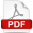Vermeer MMathematical Geodesy2015Educ127
0.1Hebisch UEbene und Sphärische Trigonometrie2005Educ68
0.6Walser HSphärische Trigonometrie. Berechnungen2002Educ23
0.1